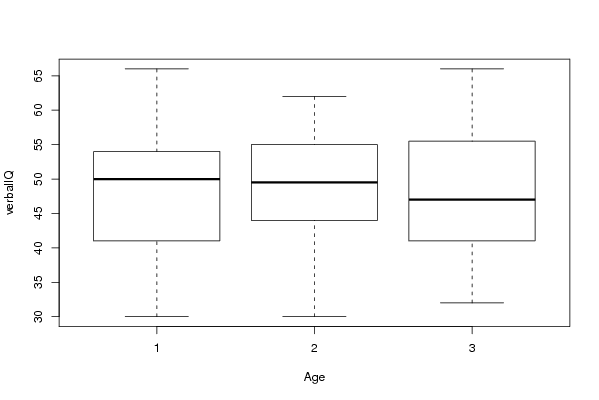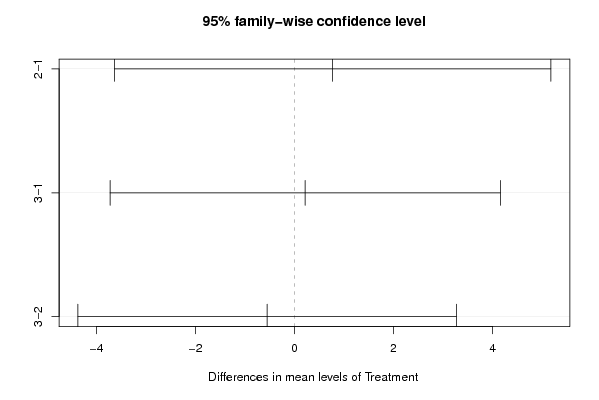## Free Statistics

of Irreproducible Research!

Author's title
Author*The author of this computation has been verified*
R Software ModuleIan.Hollidayrwasp_One Factor ANOVA.wasp
Title produced by softwareChi Square Measure of Association- Free Statistics Software (Calculator)
Date of computationThu, 03 Dec 2009 14:29:22 +0100
Cite this page as followsStatistical Computations at FreeStatistics.org, Office for Research Development and Education, URL https://freestatistics.org/blog/index.php?v=date/2009/Dec/03/t1259847020td7ld7svivm00sk.htm/, Retrieved Sat, 30 Sep 2023 12:18:20 +0000
Statistical Computations at FreeStatistics.org, Office for Research Development and Education, URL https://freestatistics.org/blog/index.php?pk=62730, Retrieved Sat, 30 Sep 2023 12:18:20 +0000
QR Codes:Original text written by user:
IsPrivate?No (this computation is public)
User-defined keywords
Estimated Impact178
Family? (F = Feedback message, R = changed R code, M = changed R Module, P = changed Parameters, D = changed Data)
-     [Chi Square Measure of Association- Free Statistics Software (Calculator)] [One Way ANOVA wit...] [2009-11-29 13:09:19] [98fd0e87c3eb04e0cc2efde01dbafab6]
-   PD  [Chi Square Measure of Association- Free Statistics Software (Calculator)] [One Way ANOVA for...] [2009-12-01 13:05:10] [3fdd735c61ad38cbc9b3393dc997cdb7]
- R P     [Chi Square Measure of Association- Free Statistics Software (Calculator)] [CARE date with Tu...] [2009-12-01 18:33:48] [98fd0e87c3eb04e0cc2efde01dbafab6]
-   PD        [Chi Square Measure of Association- Free Statistics Software (Calculator)] [comp9, 1] [2009-12-03 13:29:22] [5b58052098f4b0433c56ae9d9a4453be] [Current]
-    D          [Chi Square Measure of Association- Free Statistics Software (Calculator)] [comp 9, 2] [2009-12-03 13:38:11] [e8bb49267f0b4e611f4778412d0811f2]
-    D            [Chi Square Measure of Association- Free Statistics Software (Calculator)] [comp 3, 3] [2009-12-03 13:47:54] [e8bb49267f0b4e611f4778412d0811f2]
-    D              [Chi Square Measure of Association- Free Statistics Software (Calculator)] [comp 3, 4] [2009-12-03 13:50:36] [e8bb49267f0b4e611f4778412d0811f2]
-    D                [Chi Square Measure of Association- Free Statistics Software (Calculator)] [comp 9, 5] [2009-12-10 13:30:01] [e8bb49267f0b4e611f4778412d0811f2]
-    D                  [Chi Square Measure of Association- Free Statistics Software (Calculator)] [comp 9, 6] [2009-12-10 13:34:41] [e8bb49267f0b4e611f4778412d0811f2]
-    D                  [Chi Square Measure of Association- Free Statistics Software (Calculator)] [comp 9, 6] [2009-12-10 13:34:41] [e8bb49267f0b4e611f4778412d0811f2]
-    D                  [Chi Square Measure of Association- Free Statistics Software (Calculator)] [comp 9, 6] [2009-12-10 13:34:41] [e8bb49267f0b4e611f4778412d0811f2]
Feedback Forum

Post a new message
Dataseries X:
36	3
36	1
56	3
48	3
32	3
44	1
39	2
34	3
41	3
50	2
39	1
62	1
52	3
37	3
50	3
41	1
55	3
41	3
56	3
39	2
52	2
46	1
44	1
48	3
41	3
50	1
50	1
44	3
52	1
54	2
44	3
52	2
37	2
52	3
50	1
36	3
50	3
52	1
55	2
31	1
36	1
49	2
42	1
37	1
41	3
30	2
52	2
30	1
41	1
44	1
66	1
48	2
43	3
57	3
46	3
54	3
48	2
48	2
52	2
62	3
58	3
58	2
62	2
48	2
46	3
34	2
66	3
52	3
55	2
55	2
57	3
56	3
55	2
56	1
54	2
55	2
46	2
52	2
32	3
44	2
46	3
59	1
46	3
46	3
54	1
66	1
56	2
59	1
57	3
52	1
48	3
44	2
41	3
50	1
48	1
48	2
59	3
34	1
46	3
54	1
55	2
54	2
59	3
44	3
54	1
52	3
66	3
44	3
57	2
39	3
60	3
45	3
41	3
50	1
39	3
43	1
48	3
37	3
58	3
46	2
43	3
44	2
34	3
30	2
50	2
39	2
37	3
55	3
48	1
41	1
39	3
36	3
43	2
50	1
55	3
43	3
60	3
48	2
30	1
43	3
39	3
52	3
39	2
39	3
56	2
59	3
46	3
57	2
50	3
54	3
50	3
60	3
59	1
41	3
48	2
59	3
60	3
56	1
56	1
51	3


 Summary of computational transaction Raw Input view raw input (R code) Raw Output view raw output of R engine Computing time 2 seconds R Server 'RServer@AstonUniversity' @ vre.aston.ac.uk

\begin{tabular}{lllllllll}
\hline
Summary of computational transaction \tabularnewline
Raw Input & view raw input (R code)  \tabularnewline
Raw Output & view raw output of R engine  \tabularnewline
Computing time & 2 seconds \tabularnewline
R Server & 'RServer@AstonUniversity' @ vre.aston.ac.uk \tabularnewline
\hline
\end{tabular}
%Source: https://freestatistics.org/blog/index.php?pk=62730&T=0

[TABLE]
[ROW][C]Summary of computational transaction[/C][/ROW]
[ROW][C]Raw Input[/C][C]view raw input (R code) [/C][/ROW]
[ROW][C]Raw Output[/C][C]view raw output of R engine [/C][/ROW]
[ROW][C]Computing time[/C][C]2 seconds[/C][/ROW]
[ROW][C]R Server[/C][C]'RServer@AstonUniversity' @ vre.aston.ac.uk[/C][/ROW]
[/TABLE]
Source: https://freestatistics.org/blog/index.php?pk=62730&T=0

Globally Unique Identifier (entire table): ba.freestatistics.org/blog/index.php?pk=62730&T=0

As an alternative you can also use a QR Code:

The GUIDs for individual cells are displayed in the table below:

 Summary of computational transaction Raw Input view raw input (R code) Raw Output view raw output of R engine Computing time 2 seconds R Server 'RServer@AstonUniversity' @ vre.aston.ac.uk

 ANOVA Model verbalIQ ~ Age means 47.8 0.768 0.213

\begin{tabular}{lllllllll}
\hline
ANOVA Model \tabularnewline
verbalIQ  ~  Age \tabularnewline
means & 47.8 & 0.768 & 0.213 \tabularnewline
\hline
\end{tabular}
%Source: https://freestatistics.org/blog/index.php?pk=62730&T=1

[TABLE]
[ROW][C]ANOVA Model[/C][/ROW]
[ROW][C]verbalIQ  ~  Age[/C][/ROW]
[ROW][C]means[/C][C]47.8[/C][C]0.768[/C][C]0.213[/C][/ROW]
[/TABLE]
Source: https://freestatistics.org/blog/index.php?pk=62730&T=1

Globally Unique Identifier (entire table): ba.freestatistics.org/blog/index.php?pk=62730&T=1

As an alternative you can also use a QR Code:

The GUIDs for individual cells are displayed in the table below:

 ANOVA Model verbalIQ ~ Age means 47.8 0.768 0.213

 ANOVA Statistics Df Sum Sq Mean Sq F value Pr(>F) Age 2 13.793 6.896 0.095 0.909 Residuals 157 11404.182 72.638

\begin{tabular}{lllllllll}
\hline
ANOVA Statistics \tabularnewline
& Df & Sum Sq & Mean Sq & F value & Pr(>F) \tabularnewline
Age & 2 & 13.793 & 6.896 & 0.095 & 0.909 \tabularnewline
Residuals & 157 & 11404.182 & 72.638 &   &   \tabularnewline
\hline
\end{tabular}
%Source: https://freestatistics.org/blog/index.php?pk=62730&T=2

[TABLE]
[ROW][C]ANOVA Statistics[/C][/ROW]
[ROW][C] [/C][C]Df[/C][C]Sum Sq[/C][C]Mean Sq[/C][C]F value[/C][C]Pr(>F)[/C][/ROW]
[ROW][C]Age[/C][C]2[/C][C]13.793[/C][C]6.896[/C][C]0.095[/C][C]0.909[/C][/ROW]
[ROW][C]Residuals[/C][C]157[/C][C]11404.182[/C][C]72.638[/C][C] [/C][C] [/C][/ROW]
[/TABLE]
Source: https://freestatistics.org/blog/index.php?pk=62730&T=2

Globally Unique Identifier (entire table): ba.freestatistics.org/blog/index.php?pk=62730&T=2

As an alternative you can also use a QR Code:

The GUIDs for individual cells are displayed in the table below:

 ANOVA Statistics Df Sum Sq Mean Sq F value Pr(>F) Age 2 13.793 6.896 0.095 0.909 Residuals 157 11404.182 72.638

 Tukey Honest Significant Difference Comparisons diff lwr upr p adj 2-1 0.768 -3.637 5.174 0.911 3-1 0.213 -3.726 4.152 0.991 3-2 -0.555 -4.375 3.265 0.937

\begin{tabular}{lllllllll}
\hline
Tukey Honest Significant Difference Comparisons \tabularnewline
& diff & lwr & upr & p adj \tabularnewline
2-1 & 0.768 & -3.637 & 5.174 & 0.911 \tabularnewline
3-1 & 0.213 & -3.726 & 4.152 & 0.991 \tabularnewline
3-2 & -0.555 & -4.375 & 3.265 & 0.937 \tabularnewline
\hline
\end{tabular}
%Source: https://freestatistics.org/blog/index.php?pk=62730&T=3

[TABLE]
[ROW][C]Tukey Honest Significant Difference Comparisons[/C][/ROW]
[ROW][C]2-1[/C][C]0.768[/C][C]-3.637[/C][C]5.174[/C][C]0.911[/C][/ROW]
[ROW][C]3-1[/C][C]0.213[/C][C]-3.726[/C][C]4.152[/C][C]0.991[/C][/ROW]
[ROW][C]3-2[/C][C]-0.555[/C][C]-4.375[/C][C]3.265[/C][C]0.937[/C][/ROW]
[/TABLE]
Source: https://freestatistics.org/blog/index.php?pk=62730&T=3

Globally Unique Identifier (entire table): ba.freestatistics.org/blog/index.php?pk=62730&T=3

As an alternative you can also use a QR Code:

The GUIDs for individual cells are displayed in the table below:

 Tukey Honest Significant Difference Comparisons diff lwr upr p adj 2-1 0.768 -3.637 5.174 0.911 3-1 0.213 -3.726 4.152 0.991 3-2 -0.555 -4.375 3.265 0.937

 Levenes Test for Homogeneity of Variance Df F value Pr(>F) Group 2 1.46 0.235 157

\begin{tabular}{lllllllll}
\hline
Levenes Test for Homogeneity of Variance \tabularnewline
& Df & F value & Pr(>F) \tabularnewline
Group & 2 & 1.46 & 0.235 \tabularnewline
& 157 &   &   \tabularnewline
\hline
\end{tabular}
%Source: https://freestatistics.org/blog/index.php?pk=62730&T=4

[TABLE]
[ROW][C]Levenes Test for Homogeneity of Variance[/C][/ROW]
[ROW][C] [/C][C]Df[/C][C]F value[/C][C]Pr(>F)[/C][/ROW]
[ROW][C]Group[/C][C]2[/C][C]1.46[/C][C]0.235[/C][/ROW]
[ROW][C] [/C][C]157[/C][C] [/C][C] [/C][/ROW]
[/TABLE]
Source: https://freestatistics.org/blog/index.php?pk=62730&T=4

Globally Unique Identifier (entire table): ba.freestatistics.org/blog/index.php?pk=62730&T=4

As an alternative you can also use a QR Code:

The GUIDs for individual cells are displayed in the table below:

 Levenes Test for Homogeneity of Variance Df F value Pr(>F) Group 2 1.46 0.235 157PNG link Postscript link PDF linkPNG link Postscript link PDF link

Parameters (Session):
par1 = 1 ; par2 = 2 ; par3 = TRUE ;
Parameters (R input):
par1 = 1 ; par2 = 2 ; par3 = TRUE ;
R code (references can be found in the software module):
cat1 <- as.numeric(par1) #cat2<- as.numeric(par2) #intercept<-as.logical(par3)x <- t(x)x1<-as.numeric(x[,cat1])f1<-as.character(x[,cat2])xdf<-data.frame(x1,f1)(V1<-dimnames(y)[][cat1])(V2<-dimnames(y)[][cat2])names(xdf)<-c('Response', 'Treatment')if(intercept == FALSE) (lmxdf<-lm(Response ~ Treatment - 1, data = xdf) ) else (lmxdf<-lm(Response ~ Treatment, data = xdf) )(aov.xdf<-aov(lmxdf) )(anova.xdf<-anova(lmxdf) )load(file='createtable')a<-table.start()a<-table.row.start(a)a<-table.element(a,'ANOVA Model', length(lmxdf$coefficients)+1,TRUE)a<-table.row.end(a)a<-table.row.start(a)a<-table.element(a, paste(V1, ' ~ ', V2), length(lmxdf$coefficients)+1,TRUE)a<-table.row.end(a)a<-table.row.start(a)a<-table.element(a, 'means',,TRUE)for(i in 1:length(lmxdf$coefficients)){a<-table.element(a, round(lmxdf$coefficients[i], digits=3),,FALSE)}a<-table.row.end(a)a<-table.end(a)table.save(a,file='mytable.tab')a<-table.start()a<-table.row.start(a)a<-table.element(a,'ANOVA Statistics', 5+1,TRUE)a<-table.row.end(a)a<-table.row.start(a)a<-table.element(a, ' ',,TRUE)a<-table.element(a, 'Df',,FALSE)a<-table.element(a, 'Sum Sq',,FALSE)a<-table.element(a, 'Mean Sq',,FALSE)a<-table.element(a, 'F value',,FALSE)a<-table.element(a, 'Pr(>F)',,FALSE)a<-table.row.end(a)a<-table.row.start(a)a<-table.element(a, V2,,TRUE)a<-table.element(a, anova.xdf$Df,,FALSE)a<-table.element(a, round(anova.xdf$'Sum Sq', digits=3),,FALSE)a<-table.element(a, round(anova.xdf$'Mean Sq', digits=3),,FALSE)a<-table.element(a, round(anova.xdf$'F value', digits=3),,FALSE)a<-table.element(a, round(anova.xdf$'Pr(>F)', digits=3),,FALSE)a<-table.row.end(a)a<-table.row.start(a)a<-table.element(a, 'Residuals',,TRUE)a<-table.element(a, anova.xdf$Df,,FALSE)a<-table.element(a, round(anova.xdf$'Sum Sq', digits=3),,FALSE)a<-table.element(a, round(anova.xdf$'Mean Sq', digits=3),,FALSE)a<-table.element(a, ' ',,FALSE)a<-table.element(a, ' ',,FALSE)a<-table.row.end(a)a<-table.end(a)table.save(a,file='mytable1.tab')bitmap(file='anovaplot.png')boxplot(Response ~ Treatment, data=xdf, xlab=V2, ylab=V1)dev.off()if(intercept==TRUE){thsd<-TukeyHSD(aov.xdf)bitmap(file='TukeyHSDPlot.png')plot(thsd)dev.off()a<-table.start()a<-table.row.start(a)a<-table.element(a,'Tukey Honest Significant Difference Comparisons', 5,TRUE)a<-table.row.end(a)a<-table.row.start(a)a<-table.element(a, ' ', 1, TRUE)for(i in 1:4){a<-table.element(a,colnames(thsd[])[i], 1, TRUE)}a<-table.row.end(a)for(i in 1:length(rownames(thsd[]))){a<-table.row.start(a)a<-table.element(a,rownames(thsd[])[i], 1, TRUE)for(j in 1:4){a<-table.element(a,round(thsd[][i,j], digits=3), 1, FALSE)}a<-table.row.end(a)}a<-table.end(a)table.save(a,file='mytable2.tab')}if(intercept==FALSE){a<-table.start()a<-table.row.start(a)a<-table.element(a,'TukeyHSD Message', 1,TRUE)a<-table.row.end(a)a<-table.start()a<-table.row.start(a)a<-table.element(a,'Must Include Intercept to use Tukey Test ', 1, FALSE)a<-table.row.end(a)a<-table.end(a)table.save(a,file='mytable2.tab')}library(car)lt.lmxdf<-levene.test(lmxdf)a<-table.start()a<-table.row.start(a)a<-table.element(a,'Levenes Test for Homogeneity of Variance', 4,TRUE)a<-table.row.end(a)a<-table.row.start(a)a<-table.element(a,' ', 1, TRUE)for (i in 1:3){a<-table.element(a,names(lt.lmxdf)[i], 1, FALSE)}a<-table.row.end(a)a<-table.row.start(a)a<-table.element(a,'Group', 1, TRUE)for (i in 1:3){a<-table.element(a,round(lt.lmxdf[[i]], digits=3), 1, FALSE)}a<-table.row.end(a)a<-table.row.start(a)a<-table.element(a,' ', 1, TRUE)a<-table.element(a,lt.lmxdf[], 1, FALSE)a<-table.element(a,' ', 1, FALSE)a<-table.element(a,' ', 1, FALSE)a<-table.row.end(a)a<-table.end(a)table.save(a,file='mytable3.tab')